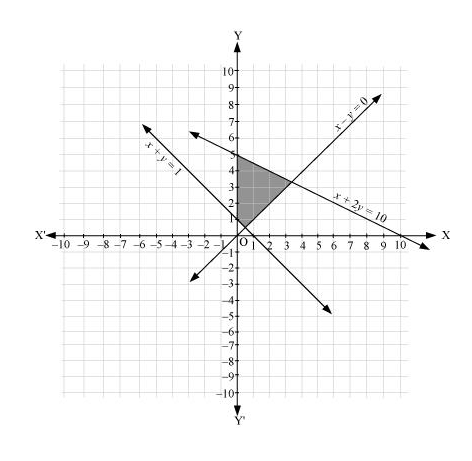# Solve the following system of inequalities graphically: x + 2y ≤ 10, x + y ≥ 1, x – y ≤ 0, x ≥ 0, y ≥ 0

Question:

Solve the following system of inequalities graphically: x + 2y  10, x + y  1, x – y  0, x  0, y  0

Solution:

$x+2 y \leq 10 \ldots$ (1)

$x+y \geq 1 \ldots$ (2)

$x-y \leq 0 \ldots$ (3)

The graph of the lines, x + 2y = 10, x + y = 1, and x – y = 0, are drawn in the figure below.

Inequality (1) represents the region below the line, x + 2y = 10 (including the line x + 2y = 10). Inequality (2) represents the region above the line, x + y = 1 (including the line x + y = 1). Inequality (3) represents the region above the line, x – y = 0 (including the line x – y = 0).

Since x  0 and y  0, every point in the common shaded region in the first quadrant including the points on the respective lines and the axes represents the solution of the given system of linear inequalities.